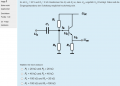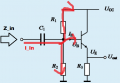# Emitterfollower / Chosing the bias divider R's

#### Tobias Hildebrandt

Joined Jan 1, 2016
44
Hello,

this is the circuit with which I am struggling. U_CC is 30V, U_out is supposed to be roughly 15V and R3 is 2kOhm. Our Prof. also requires a high input impedance.

My thoughts:
The resistors need to be of equal size, in order to achieve roughly 15V for U(out), this rules out one possible solution (R1 = 100kOhm and R2 = 20kOhm).

That left me with a problem. Isn't the input impedance calculated, h(FE) multiplied by R3? If this is the case, R1 and R2 don't play a role in input impedance and therefore I can pick up any of the three remaining results...

So, I looked around and found a different way to calculate the input impedance = U(in)/I(in). So, I was thinking, I use the 30V and divide it by R1 (20kOhm, 40kOhm or 100kOhm), this gives me the current flow through R1. Afterwards I divide 15V by R2(20kOhm, 40kOhm or 100kOhm). Finally I subtract Current I2 from I1 and I get the current running through the base(I(B)) of the transistor. Does this calculation make sense so far?
In order to calculate the impedance I divided 15V by I(B) and got the highest input impedance for the resistor pair with 100kOhm each.

According to my Prof. the right answer is 40kOhm for each resistor.

So what is wrong with my ideas? What am I missing?

Happy new year from Germany
Tobias#### Jony130

Joined Feb 17, 2009
5,439
Isn't the input impedance calculated, h(FE) multiplied by R3? If this is the case, R1 and R2 don't play a role in input impedance and therefore I can pick up any of the three remaining results...
No, Zin of a transistor + R3 is a impedance that we see when we looking into the transistor base.
Zin_T = hie + (hfe + 1)*Re ≈ hfe*R3
But input impedance of a entire amplifier is Zin =Vin/Iin ( the impedance seen by a input source)Zin = R1||R2||Zin_T

EDIT

And also don't forget that this voltage divider at transistor base is loaded by the base current (DC situation).
And Ib = Ie/(HFE + 1 ) ≈ Ie/HFE ≈ (15V/2kΩ)/HFE = 7.5mA/HFE. So in order to reduce the influence of base current on Vb voltage. The voltage divider current ( Id = Vcc/(R1+R2) ) should be larger than the base current.

Last edited:
•Tobias Hildebrandt

#### atferrari

Joined Jan 6, 2004
4,684
So in order to reduce the influence of base current on Vb voltage. The voltage divider current ( Id = Vcc/(R1+R2) ) should be larger than the base current.
Minimum 10 times, isn't it?

#### Jony130

Joined Feb 17, 2009
5,439
Minimum 10 times, isn't it?
Not always. The decision belongs to designer who knows and understands the consequences of his decision.

#### Tobias Hildebrandt

Joined Jan 1, 2016
44
Thank you for all your responses!!!

Ok, so did I get this right? Assuming h(FE) ≈ 100.

Z_inT ≈ h(FE) * R3 ≈ 100 * 2kΩ ≈ 200kΩ

1/(Z_in) = 1/200kΩ + 1/100kΩ + 1/100kΩ = 5/200kΩ ⇒ Z_in for 100kΩ = 40kΩ
1/(Z_in) = 1/200kΩ + 1/40kΩ + 1/40kΩ = 11/200kΩ ⇒ Z_in for 40kΩ = 18.2kΩ
1/(Z_in) = 1/200kΩ + 1/20kΩ + 1/20kΩ = 21/200kΩ ⇒ Z_in for 20kΩ = 9.5kΩ

I must have misunderstood something you said, because I still don't see how the voltage divider with 40kΩ produces the biggest Z_in. What am I still doing wrong?

Thanks again!
Tobias

#### Jony130

Joined Feb 17, 2009
5,439
The biggest Zin you will get if R1 = R2 = 100kΩ. But have you try include a "loading effect"? And how it will affect the Ve voltage?

#### Tobias Hildebrandt

Joined Jan 1, 2016
44
Thanks Jony! I am not 100% sure if I got your last comment, but I did a little more research and I found in "Student Manual for the Art of Electronics" by Horowitz on p.93 the following statement, it seems relevant to my problem. Horowitz writes:
"4. Let R_TH(bias) be << R_in(base); R_TH(base) ≤ 1/10 * β * 15k; beta = 100"

So, in my case I replace the 15kΩ base resistor with the 2kΩ from my Prof. β ≈ 100.

1/10 * 100 * 2kΩ ≥ R1 ιι R2 ⇒ 20kΩ ≥ R1 ιι R2.

The 100kΩ in parallel have a R(TH) of 50kΩ, the 40kΩ in parallel have a R(TH) of 20kΩ.

So this rules out the 100kΩ resistors and leaves me with the 40kΩ.

Does that argument sound legitimate?

Tobias

#### Jony130

Joined Feb 17, 2009
5,439
For R1 = R2 = 100K and HFE = 100 the Vb can be find as an approximate value:

Vb ≈ Vcc*(R2||Zin_T)/(R1 + (R2||Zin_T)) ≈ 30V * 66.7/166.7 ≈ 12V

But if we use 40kΩ resistors

Vb ≈ 13.6V

•Tobias Hildebrandt

#### Tobias Hildebrandt

Joined Jan 1, 2016
44
Thanks again! This is very helpful! This being said, this still does not explain the 40k resistors as the correct answer! Using your formula gives me for answer A (two 20k resistors) a V(b) of 14.28V. So, this would point towards answer A and I know that the 40kOhm resistors are the right answer.

Is it possible that my line of argument from post #7 is not entirely wrong?

#### Jony130

Joined Feb 17, 2009
5,439
Is it possible that my line of argument from post #7 is not entirely wrong?
You conclusion looks good, but notice that R1=R2 = 20kΩ also meet the requirement 20kΩ ≥ R1 ιι R2 --->20kΩ≥10kΩ
But know Vb is way too low. So as usually in electronics we have a trade-offs.

•Tobias Hildebrandt